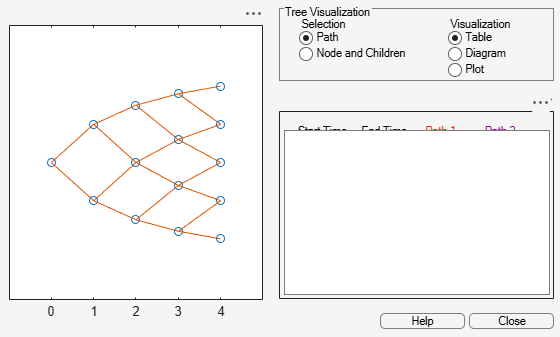## Pricing a Portfolio Using the Black-Derman-Toy Model

This example illustrates how the Financial Instruments Toolbox™ is used to create a Black-Derman-Toy (BDT) tree and price a portfolio of instruments using the BDT model.

### Create the Interest Rate Term Structure

The structure RateSpec is an interest-rate term structure that defines the initial forward-rate specification from which the tree rates are derived. Use the information of annualized zero coupon rates in the table below to populate the RateSpec structure.

` From To Rate`

`01 Jan 2005 01 Jan 2006 0.0275`

`01 Jan 2005 01 Jan 2007 0.0312`

`01 Jan 2005 01 Jan 2008 0.0363`

`01 Jan 2005 01 Jan 2009 0.0415`

`01 Jan 2005 01 Jan 2010 0.0458`

```StartDates = ['01 Jan 2005']; EndDates = ['01 Jan 2006'; '01 Jan 2007'; '01 Jan 2008'; '01 Jan 2009'; '01 Jan 2010']; ValuationDate = ['01 Jan 2005']; Rates = [0.0275; 0.0312; 0.0363; 0.0415; 0.0458]; Compounding = 1; RateSpec = intenvset('Compounding',Compounding,'StartDates', StartDates,... 'EndDates', EndDates, 'Rates', Rates,'ValuationDate', ValuationDate)```
```RateSpec = struct with fields: FinObj: 'RateSpec' Compounding: 1 Disc: [5x1 double] Rates: [5x1 double] EndTimes: [5x1 double] StartTimes: [5x1 double] EndDates: [5x1 double] StartDates: 732313 ValuationDate: 732313 Basis: 0 EndMonthRule: 1 ```

### Specify the Volatility Model

Create the structure VolSpec that specifies the volatility process with the following data.

```Volatility = [0.005; 0.0055; 0.006; 0.0065; 0.007]; BDTVolSpec = bdtvolspec(ValuationDate, EndDates, Volatility)```
```BDTVolSpec = struct with fields: FinObj: 'BDTVolSpec' ValuationDate: 732313 VolDates: [5x1 double] VolCurve: [5x1 double] VolInterpMethod: 'linear' ```

### Specify the Time Structure of the Tree

The structure TimeSpec specifies the time structure for an interest-rate tree. This structure defines the mapping between the observation times at each level of the tree and the corresponding dates.

```Maturity = EndDates; BDTTimeSpec = bdttimespec(ValuationDate, Maturity, Compounding)```
```BDTTimeSpec = struct with fields: FinObj: 'BDTTimeSpec' ValuationDate: 732313 Maturity: [5x1 double] Compounding: 1 Basis: 0 EndMonthRule: 1 ```

### Create the BDT Tree

Use the previously computed values for RateSpec, VolSpec and TimeSpec to create the BDT tree.

`BDTTree = bdttree(BDTVolSpec, RateSpec, BDTTimeSpec)`
```BDTTree = struct with fields: FinObj: 'BDTFwdTree' VolSpec: [1x1 struct] TimeSpec: [1x1 struct] RateSpec: [1x1 struct] tObs: [0 1 2 3 4] dObs: [732313 732678 733043 733408 733774] TFwd: {[5x1 double] [4x1 double] [3x1 double] [2x1 double] } CFlowT: {[5x1 double] [4x1 double] [3x1 double] [2x1 double] } FwdTree: {1x5 cell} ```

### Observe the Interest Rate Tree

Visualize the interest-rate evolution along the tree by looking at the output structure BDTTree. BDTTree returns an inverse discount tree, which you can convert into an interest-rate tree with the `cvtree` function.

`BDTTreeR = cvtree(BDTTree);`

Look at the upper branch and lower branch paths of the tree:

```%Rate at root node: RateRoot = treepath(BDTTreeR.RateTree, ) ```
```RateRoot = 0.0275 ```
```%Rates along upper branch: RatePathUp = treepath(BDTTreeR.RateTree, [1 1 1 1]) ```
```RatePathUp = 5×1 0.0275 0.0347 0.0460 0.0560 0.0612 ```
```%Rates along lower branch: RatePathDown = treepath(BDTTreeR.RateTree, [2 2 2 2])```
```RatePathDown = 5×1 0.0275 0.0351 0.0472 0.0585 0.0653 ```

You can also display a graphical representation of the tree to examine interactively the rates on the nodes of the tree until maturity. The function treeviewer displays the structure of the rate tree in the left pane. The tree visualization in the right pane is blank, but by selecting Diagram and clicking on the nodes you can examine the rates along the paths.

`treeviewer(BDTTreeR)`### Create an Instrument Portfolio

Create a portfolio consisting of two bond instruments and a option on the 5% Bond.

```% Bonds CouponRate = [0.04;0.05]; Settle = '01 Jan 2005'; Maturity = ['01 Jan 2009';'01 Jan 2010']; Period = 1; % Option OptSpec = {'call'}; Strike = 98; ExerciseDates = ['01 Jan 2010']; AmericanOpt = 1; InstSet = instadd('Bond',CouponRate, Settle, Maturity, Period); InstSet = instadd(InstSet,'OptBond', 2, OptSpec, Strike, ExerciseDates, AmericanOpt);```

Examine the set of instruments contained in the variable InstSet.

`instdisp(InstSet)`
```Index Type CouponRate Settle Maturity Period Basis EndMonthRule IssueDate FirstCouponDate LastCouponDate StartDate Face 1 Bond 0.04 01-Jan-2005 01-Jan-2009 1 0 1 NaN NaN NaN NaN 100 2 Bond 0.05 01-Jan-2005 01-Jan-2010 1 0 1 NaN NaN NaN NaN 100 Index Type UnderInd OptSpec Strike ExerciseDates AmericanOpt 3 OptBond 2 call 98 01-Jan-2010 1 ```

### Price the Portfolio Using a BDT Tree

Calculate the price of each instrument in the instrument set.

`Price = bdtprice(BDTTree, InstSet)`
```Price = 3×1 99.6374 102.2460 4.2460 ```

The prices in the output vector `Price` correspond to the prices at observation time zero (tObs = 0), which is defined as the Valuation Date of the interest-rate tree.

In the Price vector, the first element, 99.6374, represents the price of the first instrument (4% Bond); the second element, 102.2460, represents the price of the second instrument (5% Bond), and 4.2460 represents the price of the Option.